400-835-0088

APP下载excel表格是财务人员日常工作中经常会用到的工具，而掌握excel表格中的文本函数能让你在处理一些数据时事半功倍。今天理臣小编就为大家整理了一篇excel表格中经常会用到的文本函数大全，希望对大家的工作有所帮助。

1、字符转换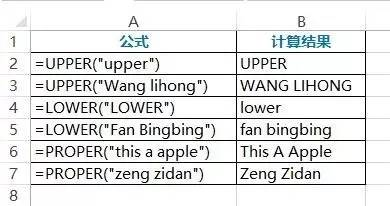01将所有字母转换成大写

=UPPER("upper")

=UPPER("Wang lihong")

02将所有字母转换成小写

=LOWER("LOWER")

=LOWER("Fan Bingbing")

03将首字母转换成大写

=PROPER("this a apple")

=PROPER("zeng zidan")

2、字符提取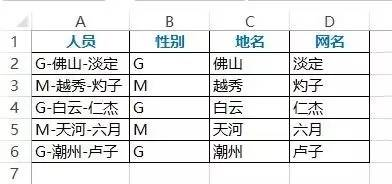01提取性别

=LEFT(A2,1)

=LEFT(A2)

02提取地名

=MID(A2,3,2)

03提取网名

=RIGHT(A2,2)

3、字符合并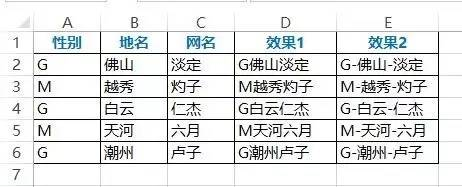01简单的合并性别、地名和网名

=PHONETIC(A2:C2)

02带分隔符号合并性别、地名和网名

=A2&"-"&B2&"-"&C2

4、字符替换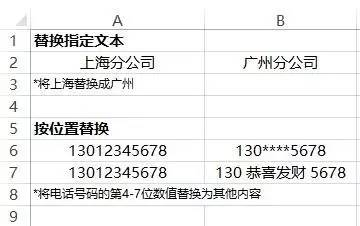01将上海替换成广州

=SUBSTITUTE(A2,"上海","广州")

02将电话号码的第4-7位数值替换为其他内容

=REPLACE(A6,4,4,"****")

=REPLACE(A7,4,4,"恭喜发财")

1、从字符串分离中文和英文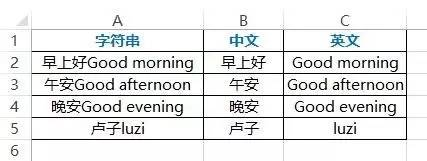01提取中文

=LEFT(A2,LENB(A2)-LEN(A2))

02提取英文

=RIGHT(A2,2*LEN(A2)-LENB(A2))

2、从字符串分离数字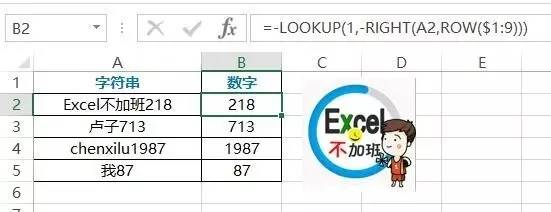01提取数字

=-LOOKUP(1,-RIGHT(A2,ROW(\$1:9)))